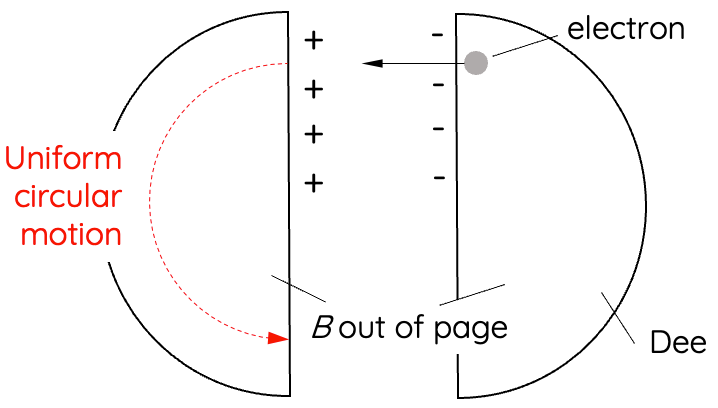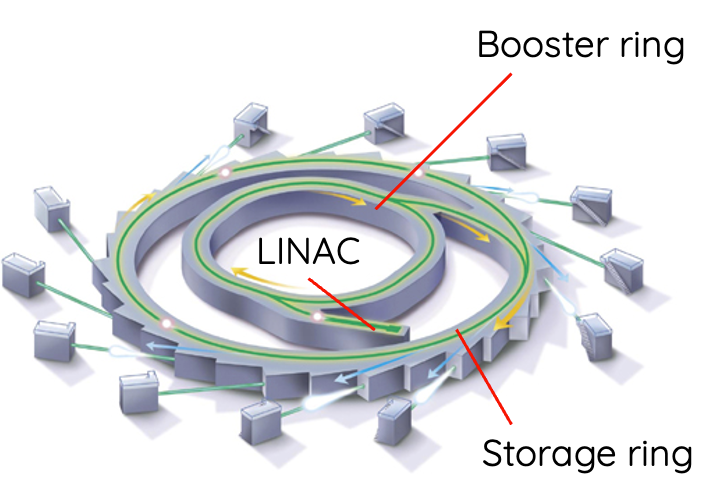# Particle Accelerators

This topic is part of the HSC Physics course under the section Deep Inside the Atom.

### HSC Physics Syllabus

• investigate the operation and role of particle accelerators in obtaining evidence that tests and/or validates aspects of theories, including the Standard Model of matter (ACSPH120, ACSPH121, ACSPH122, ACSPH146)

### Operation of Particle Accelerators

To better understand matter, physicists examine the components of the atoms. Using particle accelerators, they separate the atom into its components by inputting large amounts of energy. This enables charged particles to accelerated to very high velocities using electric fields and/or magnetic fields.

There are three main types of particle accelerators:

• Linear accelerators (LINAC)
• Cyclotrons
• Synchrotrons

### Linear Accelerators (LINAC)In a linear particle accelerator, a charged particle travels through segments (tubes) alternately polarised by AC power source. When a charged particle travels through each charged segment, the polarity of the next segment changes. As a result, an electric field is present between adjacent segments. These electric fields cause a charged particle to accelerate.

The length of each segment (tube) becomes longer further down the LINAC to accommodate for a charged particle's increasing speed and therefore, optimise its acceleration..

Linear accelerators’ lengthy structure limits the speed to which a particle can be accelerated. Thus, although it is simple in design, it is rarely used alone. It is usually used inside a synchrotron.

### CyclotronsIn a cyclotron, there are two D-shaped structures called 'dees' separated by a gap. An electric field is created in the gap by a potential difference. Magnetic fields are present in the two dees.When a charged particle travels across the gap from one dee to another, it accelerates due to the electric field. When a charged particle enters the magnetic field in one of the dees, it undergoes uniform circular motion.

When a charged particle is moving inside a magnetic field that is perpendicular to its velocity, the centripetal force is provided by the force due to the magnetic field:

$$\frac{mv^2}{r} = qvB$$

Rearranging gives:

$$r = \frac{mv}{qB}$$

According to the above equation, as the speed of a particle increases, the radius of its circular motion also increases. Thus, the trajectory of a charged particle in a cyclotron will follow a spiral outwards until it leaves the particle accelerator.

This design reduces the size of the accelerator, while still using relatively simple equipment.

### Synchrotrons

Synchrotrons are the the most modern and powerful form of particle accelerators. Synchrotrons are adapted from cyclotrons but instead consist of a closed circular loop path.Synchrotrons usually contain a LINAC which is responsible for accelerating a charged particle from rest. Once a charged particle is moving, it enters the booster ring where it accelerates due to electric and magnetic fields. The electric field in the booster ring increases the speed of the particle while the magnetic field changes its direction.

The magnetic fields in synchrotrons are synchronised with the speed of a particle in a way such that the particle's radius of circular motion remains constant. The synchronisation of the magnetic field also accounts for relativistic momentum when a particle reaches a speed at which the effects of special relativity becomes significant.

After a particle reaches a desired speed, it enters the storage ring where its acceleration produces electromagnetic radiation known as ﻿synchrotron light﻿.

### Role of Particle Accelerators

By accelerating particles to very high speeds, various concepts in physics can be tested, verified and predictions can be made.

The collision of particles and their consequent splitting has provided evidence for the Standard Model of Matter:

• Investigating the nature of fundamental matter particles. High energy collisions between electrons and protons have led to the discovery of quarks which are one of the fundamental particles in the Standard Model of Matter.
• Investigating the nature of bosons. High-energy collisions have resulted in the discovery of gluons, photons, W/Z bosons and Higgs boson. Scientists have also been able to characterise the properties of these bosons. For example, experiments on the gluon demonstrated its unique interaction with quarks and important role in binding all nucleons together in the nucleus.

Particle accelerators also play an important in other areas of physics including:

• Verifying the effects of special relativity such as time dilation, length contraction. The effects of special relativity become significant and apparent at a relativistic velocity (substantial fraction of light's speed). This is made possible in particle accelerators.
• The nature of matter at the time of the Big Bang – high energy situations can be simulated in particle accelerators. However, this method has not been successful in showing why the symmetry between matter and anti-matter production was broken during early stages of the Universe.
• The production of new radioisotopes when bombarding particles are absorbed.

Previous section: Advantages and Limitations of the Standard Model of Particle Physics